## ANALYSIS OF STRUCTURES

### Introduction

Unlike the previous chapter in this unit we will be dealing with equilibrium of supporting structure. The structures may consist of several sections. They form the supporting structures of bridges, pillars, roofs etc. It is important to have a basic knowledge of this topic as it concerns with the safety and stability of a several important structures. We will be studying about the various internal forces responsible for keeping the structures together.

Following figure gives a basic idea of what we are going to study. The given figure is a normal diagram of a book shelf. The second figure shows the role of internal forces in maintaining the system equilibrium. Free body diagram of various components are shown. It is clear from the diagram that the forces of action and reaction between various parts are equal in magnitude and opposite in direction.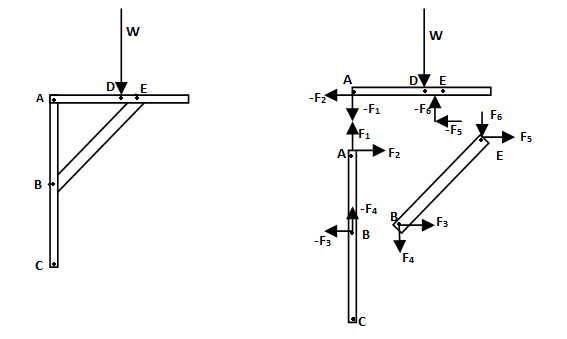### Definition of a Truss

Am truss is a network of straight slender members connected at the joints. Members are essentially connected at joints. The every member has force only at extremities. Further for equilibrium the forces in a member reduce to two force member. Thus no moments only two force member. In general trusses are designed to support. Trusses are designed to support weight only in its plane. Therefore trusses in general can be assumed to be 2-dimensional structures. Further in case weight of individual member is to be taken into consideration, half of them are to be distributed at each of the pinned ends. Figure below shows a sample truss. There are nine individual members namely DE, DF, DC, BC, BF, BA, CF, EF, FA. Structure is 2-dimensional structure, supported by pin joints at A and E.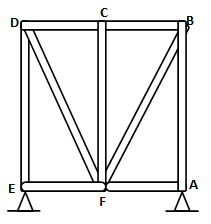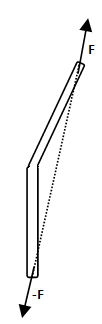### Analysis of a Truss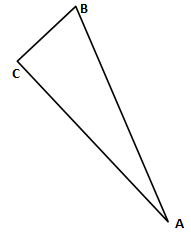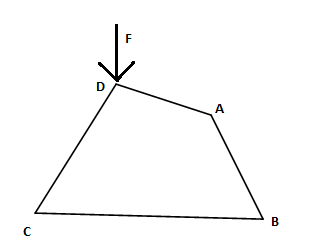The second diagram depicts that how instable the truss structure is. The truss ABCD can easily be deformed by application of the force F. Trusses constructed by adding triangles such as arms AC and CD to the above stable truss ABC are called simple trusses. No doubt simple trusses are rigid(stable). Further it is not always necessary that rigid trusses will necessary be simple. Let m be no. of members and n be number of joints. For a truss with m<2n-3 deficiency of members, unstable ,fewer unknowns than equation.
m= 2n-3 uses all the members, statically determinate
m>2n-3 excess member , statically indeterminate, more unknown than equation

### Method of Joints

In the following section we will consider about the various aspects of trusses. Distribution of forces, reactions forces at pins, tension and compression etc.

Above shown are the conditions of compression or tension, deceided as per the direction of force applied by the pin joints to the members.

Example: Find the forces in the members AF, AB , CD, DE, EC and the reactin forces at A and D. CD = 3m.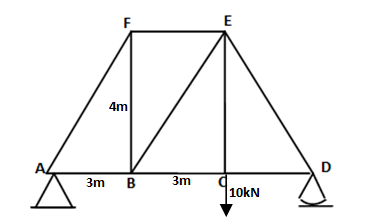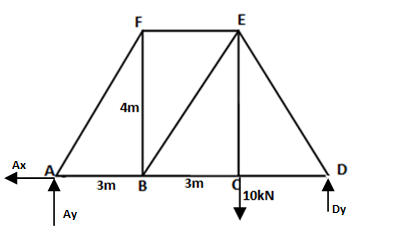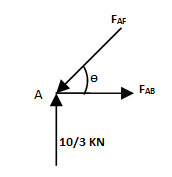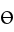### Method of Sections

As the name suggests we need to consider an entire section instead of joints. When we need to find the force in all the members, method of joint is preferrable. For finding forces in few of the specific members method of joints is preferrable. Let us consider the same diagram as before.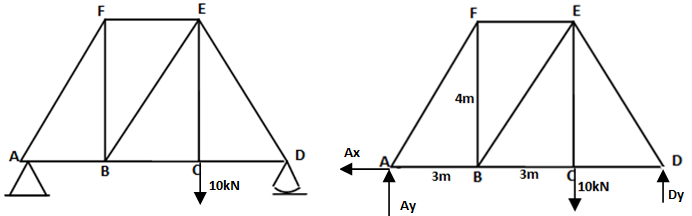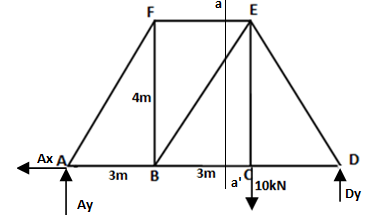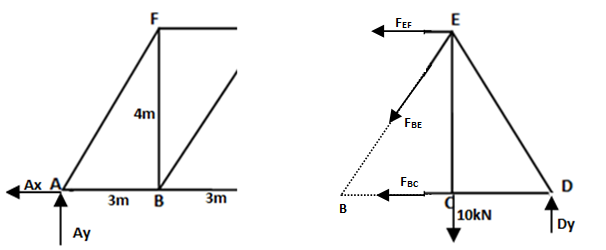## Here's a quick look at a few of the problems solved in this tutorial :

Q:
Following is a simple truss. Find the forces in the all the members by method of joints.Q:Q:
Find the reaction components at A and C. Also find the forces in each individual member, specify compression or tension. Given AD=10in ,DC=7in , BD=8in.